Author: Oscar Cronquist Article last updated on March 29, 2018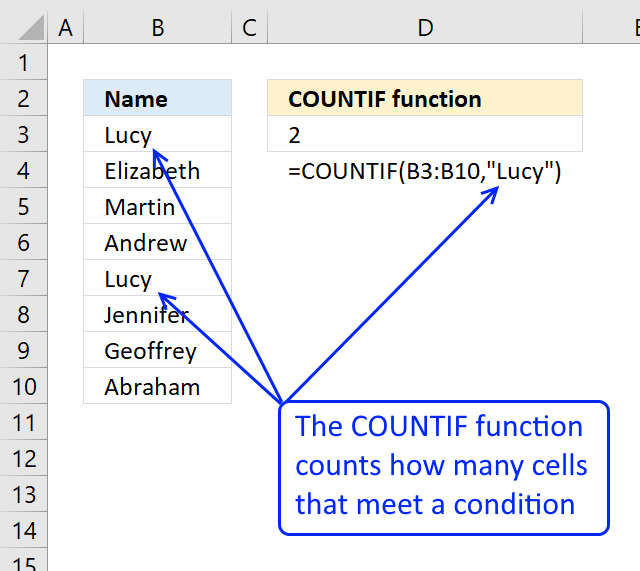The COUNTIF function calculates the number of cells that is equal to a condition.

Formula in cell D3:

=COUNTIF(B3:B10,"Lucy")

Lucy is found twice, in cell B3 and B7, the function returns 2 in cell D3.

Excel Function Syntax

COUNTIF(range, criteria)

Arguments

 range Required. The cell range you want to count the cells meeting a condition. criteria Required. The condition that you want to count.

The condition is not case sensitive.

Example 1 - Count with a single criterion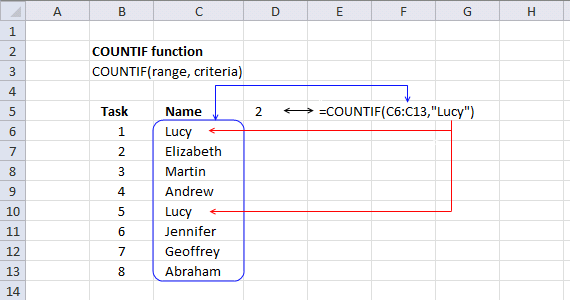The following formula in cell D5 counts the number of cells within cell range C6:C13 that meet criterion "Lucy":

=COUNTIF(C6:C13, "Lucy")

2 is returned in cell D5.

Example 2 - Count cells larger/less than a criterionThe following formula in cell D5 counts the number of cells within cell range C6:C13 that is larger than or equal to 500:

=COUNTIF(C6:C13, ">=")

Example 3 - Count cells containing a text string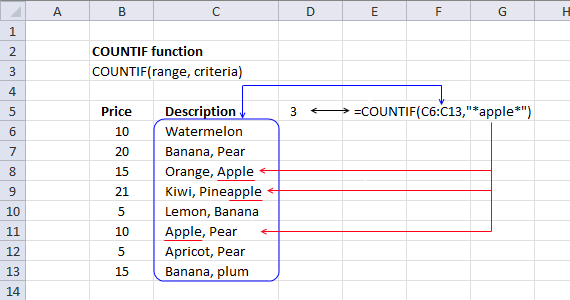The following formula in cell D5 counts the number of cells within cell range C6:C13 that contains the text string "apple":

=COUNTIF(c6:C13, "*apple*")

Example 4 - Absolute and relative cell reference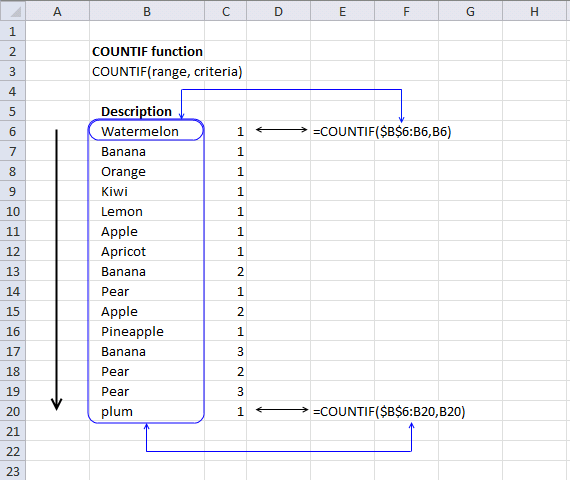Formula in cell C6:

=COUNTIF(\$B\$6:B6,B6)

\$B\$6:B6 is a cell reference to cell B6. When the cell is copied to cells below, the cell reference changes. The first part \$B\$6 i always locked to cell B6, the last part B6 changes. Cell range \$B\$6:B6 "grows" when you copy the cell.

This technique is used in this popular post: Filter unique distinct values

In cell C20:

=COUNTIF(\$B\$6:B20,B20)

becomes

=COUNTIF({"Watermelon"; "Banana"; "Orange"; "Kiwi"; "Lemon"; "Apple"; "Apricot"; "Banana"; "Pear"; "Apple"; "Pineapple"; "Banana"; "Pear"; "Pear"; "plum"},"plum") and returns 1 in cell C20.

Example 5 - Array formula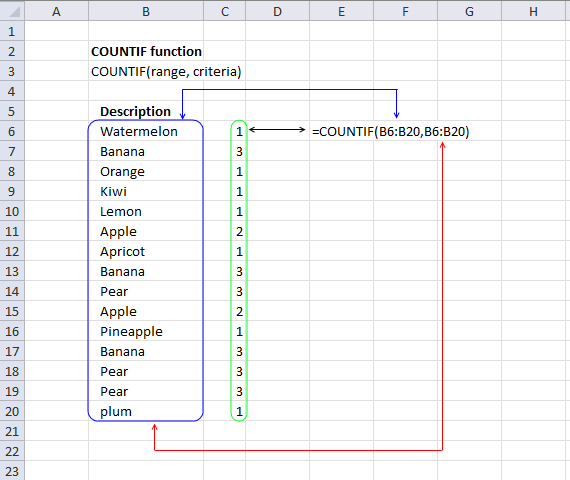Array formula in cell range C6:C20:

=COUNTIF(B6:B20, B6:B20)

How to enter an array formula

1.  Select cell range C6:C20
2. Copy / Paste formula to formula bar3. Press and hold CTRL + SHIFT
4. Press Enter
5. Release all keys

Explaining the array formula

The COUNTIF function counts the number of cells within a range that meet a single criterion. In this example I am using multiple values in the criteria argument.

Each value is in the criteria argument is used as a criterion and the returning array has the same number of values as the criteria argument.

The technique described here is used in this popular post: Count unique distinct values

=COUNTIF(B6:B20, B6:B20)

becomes

=COUNTIF({"Watermelon"; "Banana"; "Orange"; "Kiwi"; "Lemon"; "Apple"; "Apricot"; "Banana"; "Pear"; "Apple"; "Pineapple"; "Banana"; "Pear"; "Pear"; "plum"},{"Watermelon"; "Banana"; "Orange"; "Kiwi"; "Lemon"; "Apple"; "Apricot"; "Banana"; "Pear"; "Apple"; "Pineapple"; "Banana"; "Pear"; "Pear"; "plum"})

and returns {1; 3; 1; 1; 1; 2; 1; 3; 3; 2; 1; 3; 3; 3; 1} in cell range C6:C20.